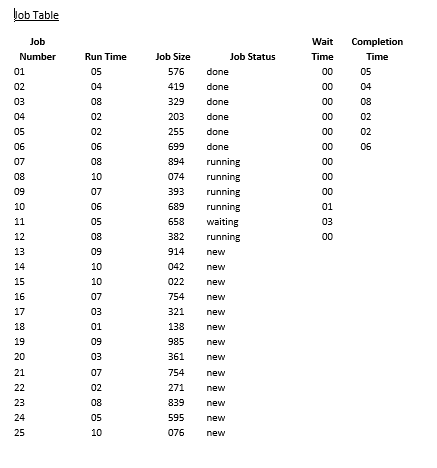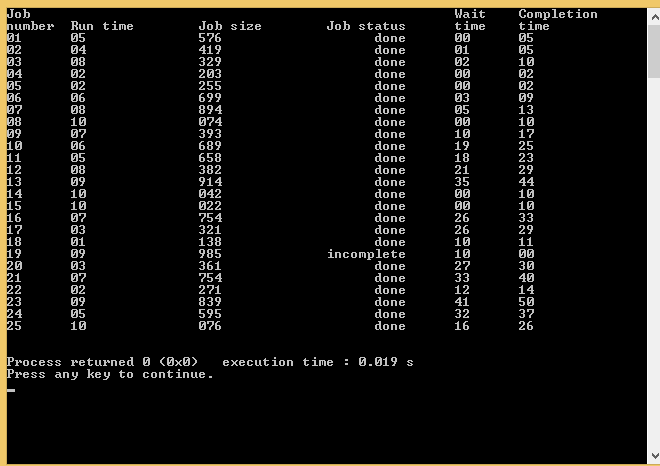# Prime factors and their applications

Having trouble understanding prime factorization? Contact us right away. We have an entire team of C assignment helpers and online tutors that can offer some assistance with this concept so you can understand it better.

## Prime Factors

### Assignment 1Solution Do you require help with C assignment, if so please consider using our service, we guarantee results or a full refund if we can't deliver.

## PrimeFactors.c

```#include

int PrimeFactors(int n, int *factors) {
int count, i;

count = 0;
for (i = 2; i <= n; i++) {
while (n % i == 0) {
/* find a prime factor, add it into the array */
factors[count] = i;
count ++;
n = n / i;
}
}

return count;
}

#define MAX_ARRAY_SIZE 100

int main() { /* test program */
int numFactors, i;
int factors[MAX_ARRAY_SIZE];

printf("Factors of 567: ");
numFactors = PrimeFactors(567, factors);
for (i = 0; i < numFactors; i++) {
printf("%d ", factors[i]);
}

printf("\nFactors of 5678901: ");
numFactors = PrimeFactors(5678901, factors);
for (i = 0; i < numFactors; i++) {
printf("%d ", factors[i]);
}
printf("\n");

return 0;
}```
Assignment 2Solution If you are looking for a C tutor or want someone to help with C assignment then we can deliver a solution.

## Emphasise.c

```#include

void Emphasise(char *word) {
int i, j, flag;

flag = 0; /* to mark whether it is between the two underscores */
for (i = 0, j = 0; word[i] != '\0'; i++) {
char c = word[i];
if (c == '_') {
flag = 1 - flag;
} else {
if (flag == 1 && c >= 'a' && c <= 'z') {
/* find a lowercase letter between two underscores,
* turn it into uppercase */
c = c - 'a' + 'A';
}
/* save back to the word */
word[j] = c;
j ++;
}
}
word[j] = '\0';
}

#define MAX_ARRAY_SIZE 100

int main() { /* test program */
char wordsA[MAX_ARRAY_SIZE] = "this is a _good_ question!";
char wordsB[MAX_ARRAY_SIZE] = "It is _over 9000_!";
char wordsC[MAX_ARRAY_SIZE] = "_Nothing to see here_";

Emphasise(wordsA);
Emphasise(wordsB);
Emphasise(wordsC);

printf("%s\n", wordsA);
printf("%s\n", wordsB);
printf("%s\n", wordsC);

return 0;
}```

## Index:

• PrimeFactors.c
• Emphasise.c
I hope that after looking at these examples you decide to contact us to offer you help with C assignment.